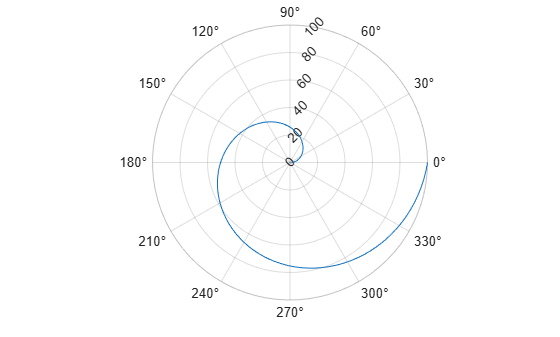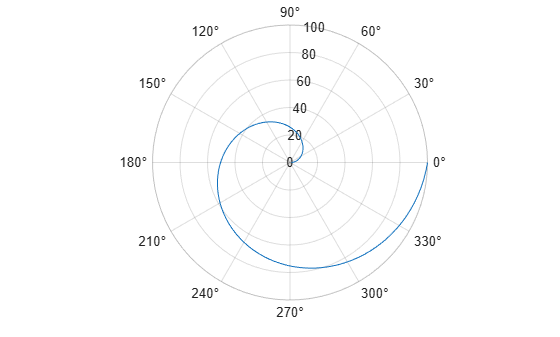# rtickangle

Rotate r-axis tick labels

## Syntax

``rtickangle(angle)``
``rtickangle(pax,angle)``
``ang = rtickangle``
``ang = rtickangle(pax)``

## Description

example

````rtickangle(angle)` rotates the r-axis tick labels for the current axes to the specified angle in degrees, where `0` is horizontal. Specify a positive value for counterclockwise rotation or a negative value for clockwise rotation.```

example

````rtickangle(pax,angle)` rotates the tick labels for the axes specified by `pax` instead of the current axes.```
````ang = rtickangle` returns the rotation angle for the r-axis tick labels of the current axes as a scalar value in degrees. Positive values indicate counterclockwise rotation. Negative values indicate clockwise rotation.```

example

````ang = rtickangle(pax)` uses the polar axes specified by `pax` instead of the current axes.```

## Examples

collapse all

Create a polar plot and rotate the r-axis tick labels 45 degrees counterclockwise.

```polarplot(1:100) rtickangle(45)```Create a polar plot and assign the polar axes object to the variable `pax`. Then, rotate the r-axis tick labels for the polar axes. Ensure that `rtickangle` affects `pax` by specifying the polar axes object as the first input argument.

```polarplot(1:100) pax = gca; rtickangle(pax,45)```Create a polar plot. Then, query the rotation angle for the r-axis tick labels. By default, the labels are not rotated.

`polarplot(1:100)``ang = rtickangle`
```ang = 0 ```

## Input Arguments

collapse all

Rotation of tick labels, specified as a scalar value in degrees, where 0 is horizontal.

Example: `rtickangle(90)`

Polar axes, or an array of polar axes. If you do not specify this argument, then `rtickangle` modifies the current axes (provided that the current axes is a polar axes object).

## Algorithms

The `rtickangle` function sets and queries the `RTickLabelRotation` property of the axes object.

## Version History

Introduced in R2016b